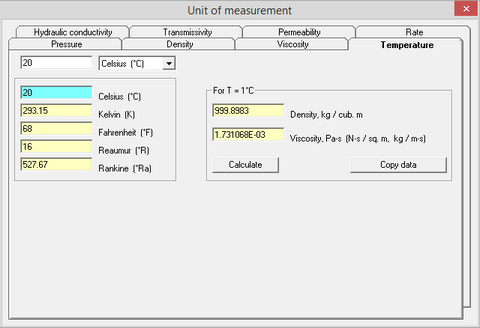﻿ Help > Unit converter (Part 1) > Temperature

# Temperature

Navigation:  Help > Unit converter (Part 1) >

# Temperature

Converts temperature to degrees of Celsius, Fahrenheit, Kelvin, Reaumur or Rankine. Calculates the density and viscosity of a liquid for the selected temperature between 0°C and 100°C (button "Calculate"). If density is entered manually, viscosity is re-calculated automatically. Pressing button "Copy data" creates a table with the three columns, temperature, density and viscosity, and copies the table on the Clipboard.Tab "Temperature" of the dialog window "Unit converter (part 1)"

Scale of Celsius (°C) Celsius

The Celsius scale is a temperature scale in SI-derived units, that is used worldwide. It is named after the Swedish astronomer Anders Celsius (1701–1744), who developed a similar temperature scale. Before being renamed to honor Anders Celsius in 1948, the unit was called centigrade, from the Latin centum, which means 100, and gradus, which means steps.

Temperature is expressed in degrees of Celsius (symbol: °C) on which temperature of melting ice is 0°C (at sea level altitude and standard atmospheric pressure) while temperature of boiling water is 100 °C (at sea level altitude and standard atmospheric pressure).

The melting and boiling points of water are no longer part of the definition of the Celsius scale. Currently, scale of Celsius is defined through scale of Kelvin, where 1°C = 1K and 0K (the absolute 0) = -273.15 °C

Scale of Fahrenheit (°F) Fahrenheit

The Fahrenheit scale was proposed in 1724 by the German physicist Daniel Gabriel Fahrenheit (1686–1736). It uses the degree Fahrenheit (symbol: °F) as the unit. Several accounts of how he originally defined his scale exist. The lower defining point, 0 °F, was established as the freezing temperature of a solution of brine made from equal parts of ice, water and a salt (ammonium chloride). Further limits were established as the melting point of ice (32 °F) and his best estimate of the average human body temperature (96 °F, about 2.6 °F less than the modern value due to a later redefinition of the scale). The scale is now usually defined by two fixed points: the temperature at which water freezes into ice is defined as 32 °F, and the boiling point of water is defined to be 212 °F, a 180 °F separation, as defined at sea level and standard atmospheric pressure.

Scale of Kelvin (K) Kelvin

The degree of Kelvin is the base unit of temperature in the International System of Units (SI). It is named after the Belfast-born, Glasgow University engineer and physicist William Thomson, 1st Baron Kelvin (1824–1907).

The Kelvin is now defined by fixing the numerical value of the Boltzmann constant k to 1.380 649×10−23 J × K−1. This unit is equal to kg×m2×s−2×K−1, where the kilogram, metre and second are defined in terms of the Planck constant, the speed of light, and the duration of the caesium-133 ground-state hyperfine transition. Thus, this definition depends only on universal constants, and not on any physical artifacts as practiced previously, such as the International Prototype of the Kilogram, whose mass diverged over time from the original value.

One Kelvin is equal to a change in the thermodynamic temperature T that results in a change of thermal energy kT by 1.380 649×10−23 J.

K = °C + 273.15

°C = K – 273.15

Scale of Reaumur (Ré)

The Réaumur scale is named after the physicist René-Antoine Ferchault de Réaumur who created it in 1731. The unit is usually abbreviated "Ré", sometimes "r". The two base points of the scale are the melting point of ice and the boiling point of water, both at normal pressure. There are 80 degrees between those values, one degree Réaumur is the 80th part of that temperature difference.

Scale of Rankine (°Ra)

The Rankine scale is a thermodynamic (absolute) temperature scale. It is based around absolute zero. Rankine is similar to the Kelvin scale in that it starts at absolute zero and 0 °Ra is the same as 0 K but is different as a change of 1 °Ra is the same as a change of 1 °F (Fahrenheit) and not 1 °C (Celsius). Note that the abbreviation °R is ambiguous, as it can also refer to the Réaumur scale.

The Kelvin and Rankine temperature scales are defined so that absolute zero is 0 kelvins (K) or 0 degrees Rankine (°R). The Celsius and Fahrenheit scales are defined so that absolute zero is −273.15 °C or −459.67 °F.